# 编译

SonderLau ... 2020-12-24 Notes
• 编译原理

# # 编译

## # 绪论

### # 课程主要内容

• 编译的基本理论
• 形式文法 形式语言
• 编译器实现的技术
• 编程实现一个小型语言的编译器

### # Q & A

1. 编译器使用 C 语言作为目标语言的好处是什么？

C 语言比较底层一些 编译器能够编译并使用到几乎所有的硬件上

For the C language there are many compilers available that compile to almost every hardware.

2. 编译器和解释器的区别是什么？

编译器是用来读取一种程序的语言(源语言) 然后把他翻译成另一种语言的相等的程序(目标语言) 并报告在转换过程中发现的任何错误

解释器直接处理用户输入的源程序符号

A compiler is a program that can read a program in one language - the source language - and translate it into an equivalent program in another language – the target language and report any errors in the source program that it detects during the translation process.

Interpreter directly executes the operations specified in the source program on inputs supplied by the user.

### # 编译器的结构

• 分析 Analysis
• 把源程序分解为多个组成要素 并加入语法结构
• 收集有关信息 并存放在符号表的数据结构中
• 也称为 前端 Front End
• 综合 Synthesis
• 根据中间 表示和符号表中的信息来构造用户期待的目标程序
• 也称为 后端 Back End

#### # 词法分析 Lexical Analysis

<token-name, attribute-value>

1
• token-name
• 由语法分析使用的抽象符号
• attribute-value
• 指向符号表中关于这个词法单元的条目

e.g. position = initial + rate * 60

• position
• 词素
• <id, 1>
• =
• 词素
• < = >
• initial
• 词素
• <id, 2>
• +
• 词素
• <id, 3>
• rate
• 词素
• <id, 3>
• *
• 词素
• < * >
• 60
• 词素
• <number, 4>

<id, 1> < = > <id, 2> < + > <id, 3> < * > <number, 4>

### # 语法分析 Syntax Analysis### # 中间代码生成 Intermediate Code Generation

• 三地址码 Three address-code

t1 = inttofloat(60)

t2 = id3 * t1

t3 = id2 + t2

id1 = t3

### # 遍 Pass

• 遍数多
• 结构清晰 但是时间效率不够高
• 遍数少
• 编译速度快 但是对机器的内存要求高• 取一个编译器的前端 重写他的后端就产生了让同一种语言在另一个机器上的编译器
• 不同的前端用同一个后端 就达到了一个机器上多个编译器

### # 自展技术### # 编译器的伙伴们

#### # 预处理器 Pre-processors

• 删除注释
• 宏展开
• 将多个分开的源文件进行聚合

#### # 汇编器 Assemblers

• 处理汇编语言代码 产生可重定位的机器代码

## # 文法与语言

### # 字母表 Alphabet

$\sum$ 是一个 有穷符号集合

e.g. 二进制字母表 ASCII 字符集 Unicode 字符集

#### # 字母表的运算

##### # 乘积 Product

$\sum_1 \sum_2 = \{ ab | a \in \sum_1 , b \in \sum_2 \}$

#### # n 次幂 Power

$\sum^0 = { \epsilon }$

$\sum ^n = \sum^{n-1} \sum , n\geqslant 1$

##### # 正闭包 Positive closure

$\sum ^ + = \sum \cup \sum^2 \cup \sum^3 \cup ...$

##### # 克林闭包 Kleene closure

$\sum ^* = \sum^0 \cup \sum^+$

### # 串 String

$\sum$ 是一个字母表 $\forall x \in \sum^*$ 则 x 称为是 $\sum$ 上的一个

• 串是字母表中符号的一个有穷序列
• 串的长度 记作 $|s|$
• 指的是 s 中符号的个数
• 空串 是长度为 0 的串 用 $\epsilon$ 表示
• $|\epsilon| = 0$
• 前缀 Prefix
• 移走符号串尾部的 0 个或多个符号得到的符号串
• 后缀 Suffix
• 删去符号串头部的 0 个或多个符号得到的符号串
• 子串
• 删去一个前缀和一个后缀得到的符号串

#### # 串的运算

##### # 连接 Concatenation

$\epsilon s = s \epsilon = s$

### # 文法的形式化定义

$G = (V_T , V_N , P , S)$

• $V_T$ 终结符号集

• 文法定义的语言的基本符号
• $V_N$ 非终结符号集

• 是用来表示语法成分的符号
• 表示一个中介符号串的集合
• $P$ 产生式集合

• 描述了将终结符和非终结符组合成串的方法

$\alpha \rightarrow \beta$

$\alpha \in (V_T \cup V_N) ^ +$ 至少包含$V_N$ 中的一个元素

$\beta \in (V_T \cup V_N) ^*$

• $S$ 开始符号

• 指定某非终结符号是开始符号

$\alpha \rightarrow \beta_1 | \beta_2 | \beta_3 | ... | \beta_n$

### # 推导 Derivation 与 规约 Reduction

#### # 直接推导与直接规约• $\alpha \overset{0}{\Rightarrow} \alpha$
• $\overset{+}{\Rightarrow}$ 表示经过整数步的推导
• $\overset{*}{\Rightarrow}$ 表示经过若干步(可以为 0)步推导

### # 句型 和 句子

$if \; S \overset{*}{\Rightarrow} \alpha ,\alpha \in (V_T \cup V_N)^*$

$\alpha$$G$的一个句型 sentential form

• 一个句型中可以包含终结符 也可以包含非终结符 也可以是空串

$if \; S \overset{*}{\Rightarrow} w , w \in V_T ^*$$w$$G$ 的一个句子 sentence

• 句子是不包含非终结符的句型

### # Chomsky 文法分类体系

#### # 0 型文法 Type-0 Grammar

$\forall \alpha \rightarrow \beta \in P$ 推导式左侧至少含有 1 个非终结符

0 型语言

#### # 1 型文法 Type-1 Grammar

$\forall \alpha \rightarrow \beta \in P, |\alpha| \leq |\beta|$

#### # 2 型文法 Type-2 Grammar

$\forall \alpha \rightarrow \beta \in P , \alpha \in V_N$

#### # 3 型文法 Type-3 Grammar

$Right \ Linear : A \rightarrow wB \enspace or \enspace A \rightarrow w \\ Left \ Linear : A \rightarrow Bw \enspace or \enspace A \rightarrow w$

#### # 四种文法的关系

• 0 型文法
• 产生式左侧至少包含 1 个非终结符
• 1 型文法
• $|\alpha| \leq | \beta|$
• 2 型文法
• $\alpha \in V_N$
• 3 型文法
• 左线性 右线性• 0 型语言
• 图灵机
• 1 型语言
• 有界图灵机
• 线性有界自动机
• 2 型语言
• 下推自动机
• 3 型语言
• 有限自动机

### # 分析树

• 根节点的标号为 文法开始符号
• 内部节点标识 为 非终结符号
• 叶结点的标号既可以是非终结符 也可以是终结符
• 一次推导对应一颗分析树
• 一颗分析树可能对应若干推导

### # 短语

• 如果子树只有父子两代节点 那么这课子树的边缘称为该句型的一个 直接短语## # 词法分析

### # 词法分析器的作用

• 单词符号 token
• 词法分析器的输出形式 二元式 < 类别,属性值 >
• 模式 pattern
• 描述一个单词符号的可能形式
• 词素 lexeme
• 源程序中的一个字符序列 是单词符号的实例

### # 有限自动机

• 有限自动机是 识别器recognizer 它们只能对每个可能的输入串简单地回答 是 或 否
• 分类
• 不确定的有穷自动机 NFA
• 对其边上的标号没有任何限制
• 一个符号标记离开同一状态的多条边 且$\epsilon$ 也可以作为标号
• 确定的有穷自动机 DFA
• 有且仅有一条离开该状态 以该符号为标号的边

NFA 与 DFA 能识别的语言集合是相同的

#### # 不确定的有限自动机 NFA

NFA 由以下几部分组成

• 有穷的状态集合 $S$
• 一个输入符号集合 $\sum$ 即输入字母表 假设$\epsilon$ 不是 $\sum$ 中的元素
• 一个转换函数 transition function 为每个状态和 $\sum \cup \{ \epsilon \}$中的每个符号都给出了相应的后继状态next state的集合
• $S$中的一个状态$s_0$被指定为开始状态 或者说 初始状态
• $S$ 的一个子集 $F$ 被指定为接受状态 或者说 终止状态的集合

$(a|b)*abb$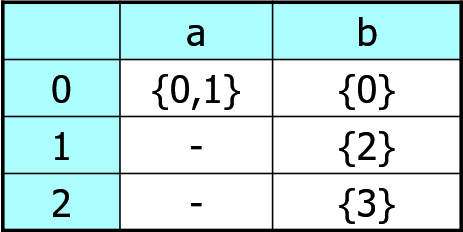NFA 能识别的所有字符串构成的集合称为 NFA 定义的语言

#### # 确定的有限自动机 DFA

• 没有 $\epsilon$ 边转移
• 一个状态面临一个输入符号时最多只转移到一个状态

#### # NFA - DFA 的转换

$\epsilon - closure (s)$

$\epsilon - closure (T)$

$Move(T,a)$

e.g#### # 从正则表达式构造 NFA

1. $\epsilon$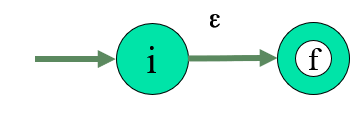2. $a$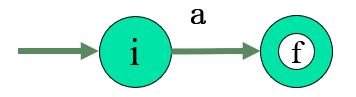3. $s | t$4. $st$5. $s^*$#### # DFA 的化简 \ 最小化

• 为 DFA 寻找一个状态数比较少的等价 DFA
• 任何 DFA （或 NFA）都存在（唯一）一个状态数最少的 DFA 与之等价
##### # 求同法
• 基本思想
• 寻找等价状态 合并
• 等价状态必须满足两个条件
• 一致性条件
• 状态 s 和 t 必须同时为接受状态或非接受状态
• 蔓延性条件
• 对于所有的输入符号,状态 s 和 t 必须转移到等价的状态中
##### # 求异法
• 首先把 DFA 的状态集划分为非接受状态组和接受状态组,作为初始划分 П

• 执行以下过程

• 最初,令 Пnew= П; for( 对于 П 中的每个组 G ) { 将 G 划分为更小的组,使得两个状态 s 和 t 在同一个小组中,当且仅当对于所有的输入符号 a,状态 s 和 t 都能转换到达 П 中的同一组; 在 Пnew 中,将 G 替换为对 G 进行细化的那些小组; }

• 如果 Пnew= П,则令 Пfinal = П 并执行 4；否则用 Пnew替换 П 并重复执行 第二步 .

• 划分 Пfinal 中的每个组内的状态均是等价的,作为一个新状态

• 用正规式表示
• 用正规文法产生
• 用有限自动机识别

## # 语法分析

### # 语法分析的概述

• 是 给出分析树
• 否 指出错误出现的位置和错误的性质

### # 自顶向下分析 Top-down parsing

#### # 递归下降法 - 不确定的自顶向下分析

• 优点
• 对文法的限制小
• 缺点
• 回溯 效率底下 代价高
• 出错时难以确定出错的位置

#### # First 集

• X 是终结符号 FIRST(X) = { X }
• $X \rightarrow \epsilon$ 则把$\epsilon$ 加入到 FIRST(X)
• $X \rightarrow Y_1Y_2\dots Y_k$
• $FIRST (Y_1) - \{ \epsilon \}$ 放入到 FIRST(X)
• 如果 $Y_1 \overset{*}{\Rightarrow}\epsilon$ 则把 $FIRST (Y_2) - \{ \epsilon \}$ 加入到 FIRST(X)
• 如果 $Y_1 Y_2 \overset{*}{\Rightarrow} \epsilon$ 则把 $FIRST (Y_3) - \{ \epsilon \}$ 加入到 FIRST(X)
• ....
• 如果 $Y_1Y_2 \dots Y_{k-1} Y_{k} \overset{\epsilon}{\Rightarrow}$ 则把 $\epsilon$ 加入到 FIRST(X)

e.g.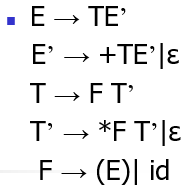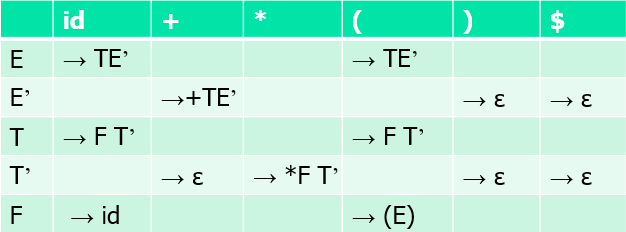#### # 非 LL(1) 文法 到 LL(1) 文法的等价转换

##### # 提取左因子 Left Factoring

$A \rightarrow a\beta | a\gamma$

$A \rightarrow a\beta_1 | a\beta_2 | a\beta_3|\dots|a\beta_n \\ A \rightarrow a (\beta_1 | \beta_2 | \beta_3 | \dots |\beta_n ) \\ A \rightarrow a B \\ B \rightarrow \beta_1 | \beta_2 | \beta_3 | \dots |\beta_n$

##### # 消除左递归 Left Recursion

$A \rightarrow Aa | \beta$ 的产生式或 $A \Rightarrow ^+ Aa$ 的推导

###### # 直接左递归

$A \rightarrow Aa_1 |Aa_2 |\dots|Aa_m| \beta_1 | \beta_2 | \beta_3 | \dots |\beta_n$

$A \rightarrow \beta_1 B |\beta_2 B |\dots |\beta_n B | \\ B \rightarrow a_1 B | a_2 B | \dots |a_n B | \varepsilon$

###### # 间接左递归

$A \Rightarrow ^ + A a$ 的推导

$A_1A_2A_3\dots A_n$

for ( 从 1 到 n 的每个 i ) {

​ for ( 从 1 到 i-1 的每个 j ) {

​ 将每个形如 $A_i \rightarrow A_j\gamma$的产生式替换为产生组

$A_i \rightarrow \sigma_1 \gamma | \sigma_2 \gamma | \sigma_3 \gamma | \dots | \sigma_k \gamma$

​ 其中 $A_j \rightarrow \sigma_1 | \sigma_2 | \sigma_3| \dots | \sigma_k \gamma$ 是所有 $A_j$ 的产生式

​ }

​ 消除 $A_i$ 它产生式之间的立即左递归

}

### # 自底向上分析 Bottom-up parsing

• 每一步都是将最右推导得到的句型的最左可规约串规约为产生式的左部符号
• 分析过程是寻找一个最左规约序列的过程
• 输出的是一个产生式序列

• 对输入符号串自左向右进行扫描 并将输入符逐个移入一个 First-in-Last-out 栈的结构
• 若栈顶符号串是某个句型的可规约串 就用相应的产生式的 左部非终结符代替
• 重复这一过程 直到只剩下开始符号

• 在右句型中寻找可规约串
• 不同的寻找可规约串的方法 形成了不同的自底向上分析法

### # 算符优先分析

• a =· b
• 当且仅当 G 中含有如 $A \rightarrow \dots ab \dots$ 或者 $A \rightarrow \dots aBb \dots$ 的产生式
• a <· b
• 当且仅当 G 中含有形如 $A \rightarrow \dots aB \dots$ 的产生式 且有 $B \overset{*}{\Rightarrow} b \dots$ 或者 $B \overset{*}{\Rightarrow} Cb$
• a ·> b
• 当且仅当 G 中含有形如 $A \rightarrow \dots Bb \dots$ 的产生式 且有 $B \overset{+}{\Rightarrow} \dots a$ 或者 $B \overset{+}{\Rightarrow} \dots aC$

#### # 算符优先关系表的构造

• 由定义直接构造

• 通过计算 FIRSTVT集 与 LASTVT 集 构造

• $FIRSTVT(B) = \{ b| B \overset{+}{\Rightarrow} b \dots \; or \; B \overset{+}{\Rightarrow} Cb \dots \}$
• 对于非终结符 B 其往下推导所可能出现的首个终结符
• $LASTVT(B) = \{ a | B \overset{+}{\Rightarrow} \dots a \; or \; B \overset{+}{\Rightarrow} \dots aC \}$
• 对于非终结符 B 其往下推导所可能出现的最后一个终结符
• a =· b
• 直接看产生式的右部 $A \rightarrow \dots ab \dots$$A \rightarrow \dots aBb$a =· b
• a <· b
• 求出每个非终结符 B 的 FIRSTVT(B)
• $A \rightarrow \dots aB \dots$$\forall b \in FIRSTVT(B) ,a <· b$
• a ·> b
• 求出每个非终结符 B 的 LASTVT(B)
• $A \rightarrow \dots Bb \dots$$\forall a \in LASTVT(B), a·>b$

a 和 b 之间的优先关系 与 b 和 a 之间的优先关系是根本不同的

• 从左端开始扫描串直到遇到第一个 ·> 为止

• 向左扫描 跳过所有 =· 直到遇到第一个 <· 为止

• 句柄包括从第二步遇到的<·的右部到第一个>·的左部之间的所有符号

包括介于其间或者两边的非终结符号

#### # 优先函数 Precedence Functions

• 在实现算符优先分析时 往往不用算符优先关系表 而是两个优先函数

• a <· b
• f(a) < g(b)
• a =· b
• f(a) = g(b)
• a ·> b
• f(a) > g(b)
• 优先函数的优点

• 节约存储空间
• 便于执行比较运算
• 优先函数的缺点

• 损失了错误检测能力
• 并不是每个优先关系表都存在优先函数
• 算符优先分析法的出错处理

• 在以下两种情况下发现错误

• 栈顶终结符号与当前输入符号无优先关系

• 在优先关系表的空白处填入错误处理子程序的入口指针

•  插入 id 输出信息 缺少运算对象
• $) : 删除 ) 输出信息 右括号不匹配 • idid 或 )( : 插入运算符 + 输出信息 缺少运算符号 • ($ : 弹出 ( 输出信息 缺少右括号
• 归约时没有产生式的右部与可归约串匹配

• 若包含 + - * . ↑ 的可归约串被归约 检查两端是否出现非终结符号 (E 表达式) 若否 出错 输出 缺少运算对象
• 若归约 id检查两端是否出现非终结符号 若有 出错 输出 缺少运算符号
• 若归约 () 检查括号之间是否有一个非终结符号 若无 出错 输出 括号之间无表达式

#### # 算符优先分析法的局限性

• 不是规范规约 得不到完整的分析树
• 适用范围窄 一般的语言很难满足算符优先文法的要求 只能用来分析表达式
• 更一般的自底向下分析法是 LR 分析法

### # LR 分析

LR (k)分析技术

• L
• 从左到右扫描输入串
• R
• 构造一个最右推导的逆过程
• 即最左规约
• k
• 在做出分析决定前（即构造分析表）时要向前看的输入符号个数
• 通常为 0 或 1

LR 分析技术是功能最强的（自底向上）语法分析技术

LR 分析器的逻辑结构• LR 分析程序
• 固定不变的 对于所有 LR 分析器都是一样的
• 分析表
• 是 LR 分析法的核心 由两部分组成
• 不同的 LR 分析器 具有不同的分析表
• LR(0) SLR(1) LR(1) LALR(1)
• 保存状态符号和文法符号
• $s_i$ 状态 $X_i$ 文法符号
• 输入缓冲
• 输出
• 产生式序列
• LR 分析方法是当前最广义的无回溯的 移进 - 归约方法
• 根据栈中的符号串和向右顺序查看输入串中的 $k(k\geq0)$个符号 就能唯一确定分析器的动作是移进还是归约 以及用哪个产生式进行归约
• LR(k) 分析技术是 Knuth 于 1965 年首先提出来的
• 优点
• 使用范围广 分析速度快 报错准确

#### # 分析表

• 分析表由 ACTION 表和 GOTO表两部分组成
• $ACTION[s_m,a_i]$表示当状态 sm 面临输入 ai 时的动作
• 动作包括主要包括四种
• $SHIFT s$$a_i$$s=ACTION[s_m,a_i]$分别压入符号栈中
• $REDUCE \ A \rightarrow \beta$ 产生归约
• $ACCEPT$ 接受 停机 成功
• $ERROR$ 报错 调用出错处理
• $GOTO[s_m,X_i]$ 表示当状态 $s_m$遇到文法符号$X_i$时需要跳转到的下一状态

#### # 分析器运行

• 根据当前栈顶状态符号与输入符号查分析表决定下一步动作
• 把栈中符号序列和剩余输入串看成一个二元式格局,初始格局表示为 $( s_0,a_1a_2 \dots a_n )$
• 假设当前 $(s_0 X_1 X_2 s_2 \dots X_m s_m, \; a_ia_{i+1} \dots a_n \)$
• $ACTION[s_m,a_i] = shift \; s$
• 二元式变为 $(s_0 X_1 X_2 s_2 \dots X_m s_ma_is, \; a_{i+1}\dots a_n \)$
• $ACTION[s_m,a_i] = reduce \; A \rightarrow \beta$
• 假设 $|\beta| = r$ 则二元式变为 $(s_0 X_1 X_2 s_2 \dots X_{m-r} s_{m-r} As, a_ia_{i+1}\dots a_n \)$
• 其中 $s = GOTO[s_{m-r},A] , \beta = X_{m-r}X_{m-r+2}\dots X_m$

LR 分析的关键问题是如何构造分析表 不同的构造方法形成不同的分析方法

LR 分析过程中的性质与特点

• 栈中的文法符号串并上剩余的输入串构成一个右句型
• 当该右句型出现在栈顶时 归约 否则移进
• 栈中的文法符号串是当前句型的前缀 该不包含句型句柄后面的符号 称之为 活前缀 Viable Prefixes